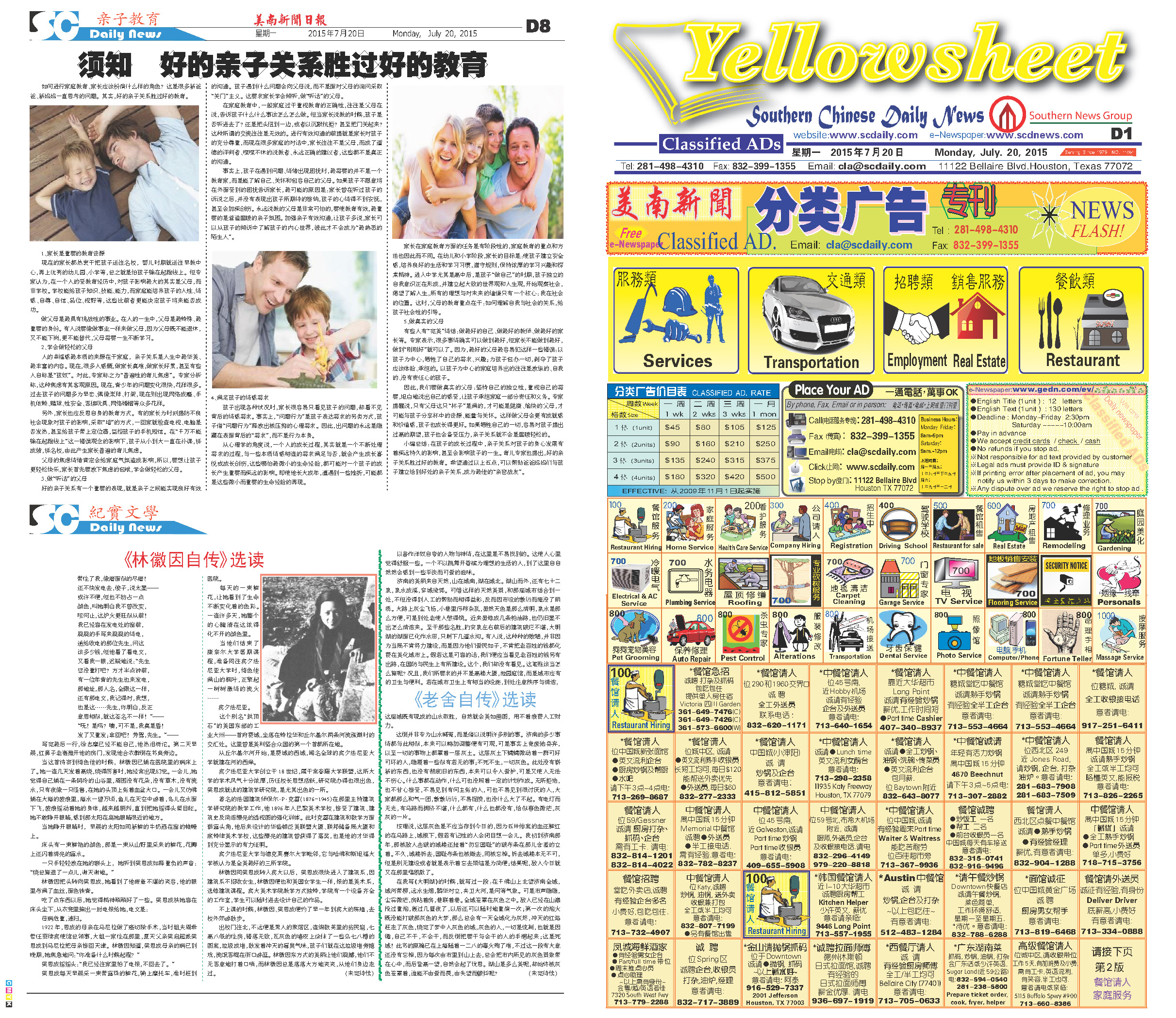150730 Epaper

 A Section B SectionC Section D Section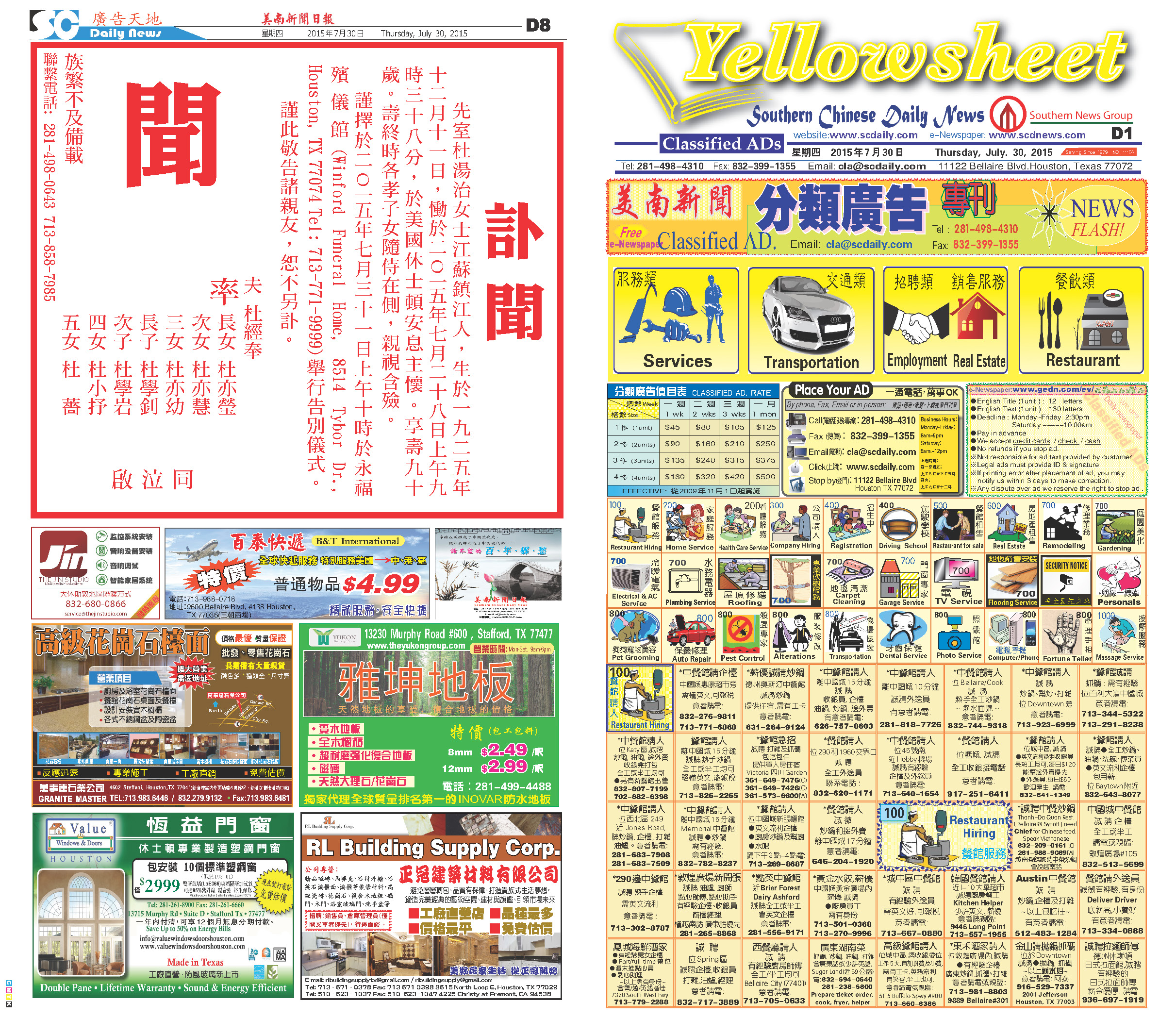150729 Epaper

 A Section B SectionC Section D Section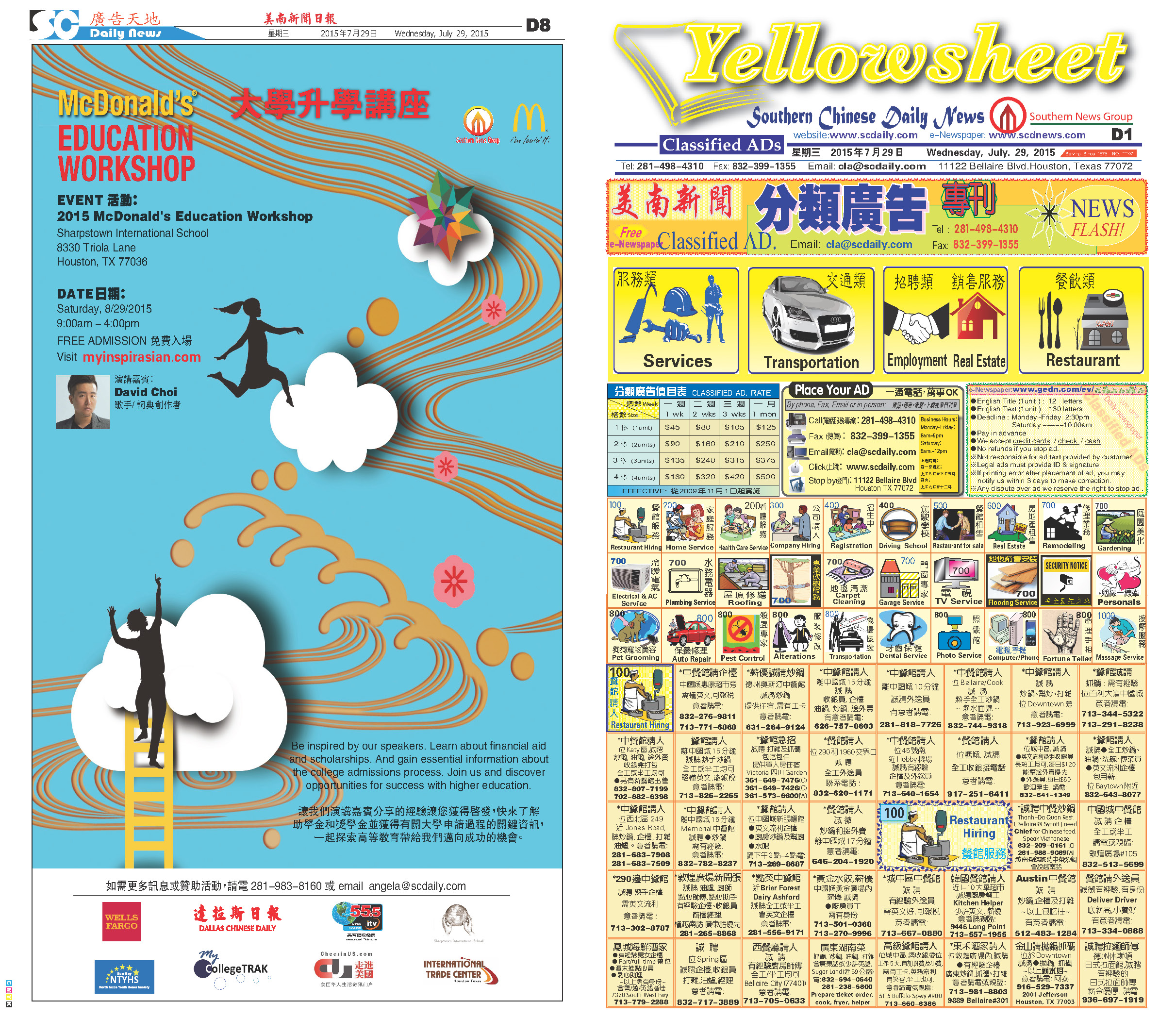150728 Epaper

 A Section B SectionC Section D Section150727 Epaper

 A Section B SectionC Section D Section150726 Epaper

 A Section B SectionC Section D Section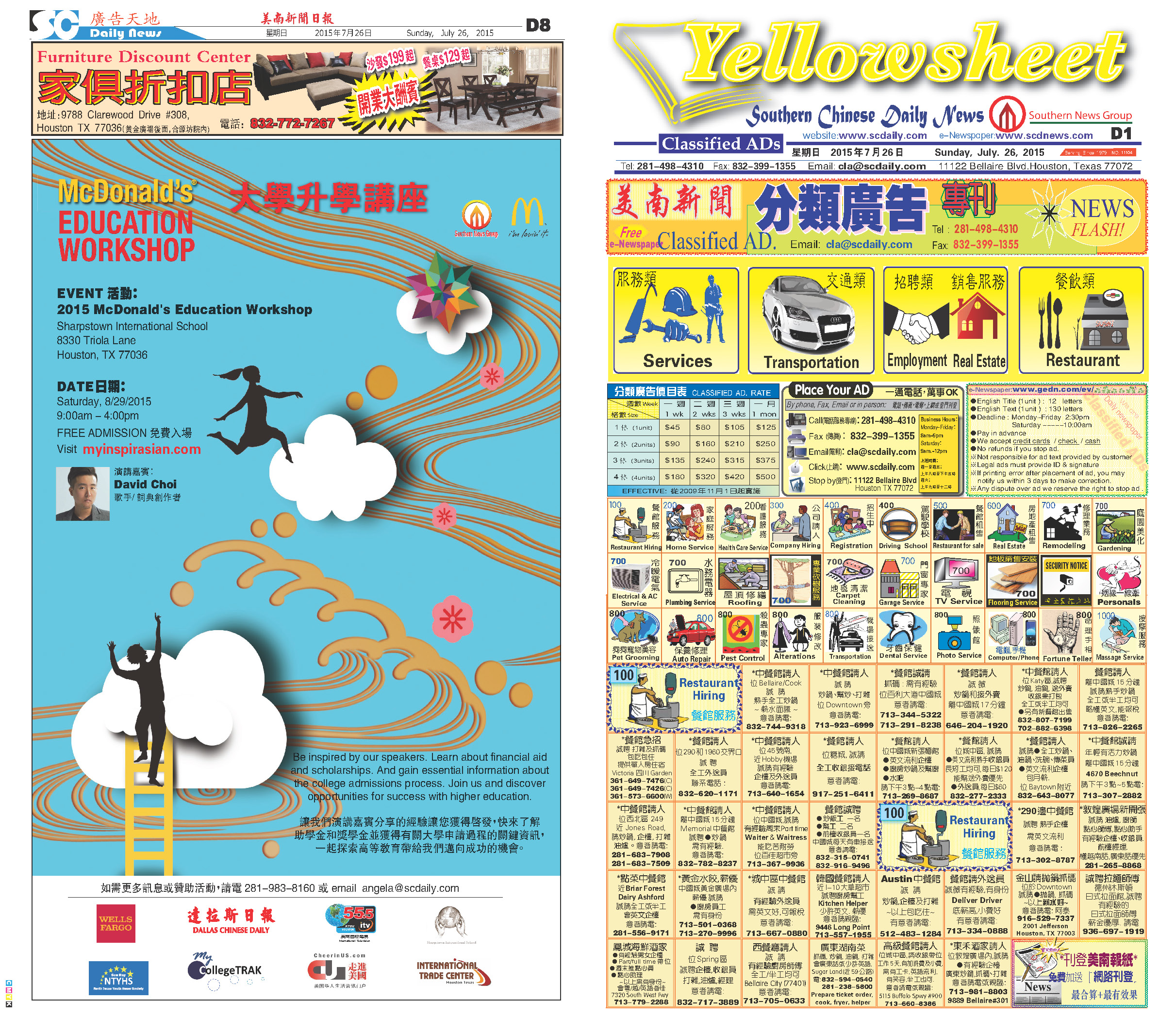150725 Epaper

 A Section B SectionC Section D Section150724 Epaper

 A Section B SectionC Section D Section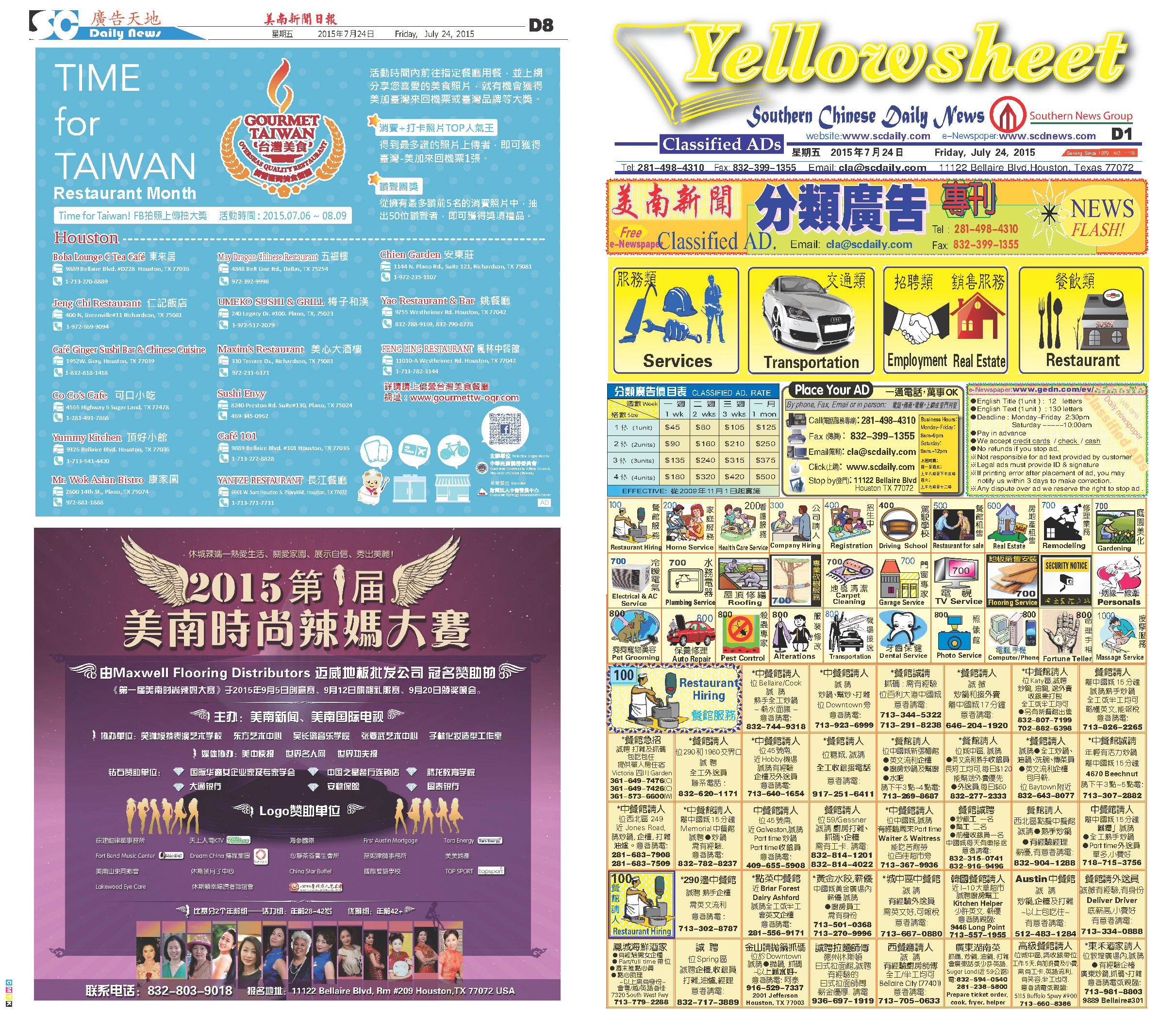150723 Epaper

 A Section B SectionC Section D Section150721 Epaper

 A Section B SectionC Section D Section150722 Epaper

 A Section B SectionC Section D Section150720 Epaper

 A Section B SectionC Section D Section